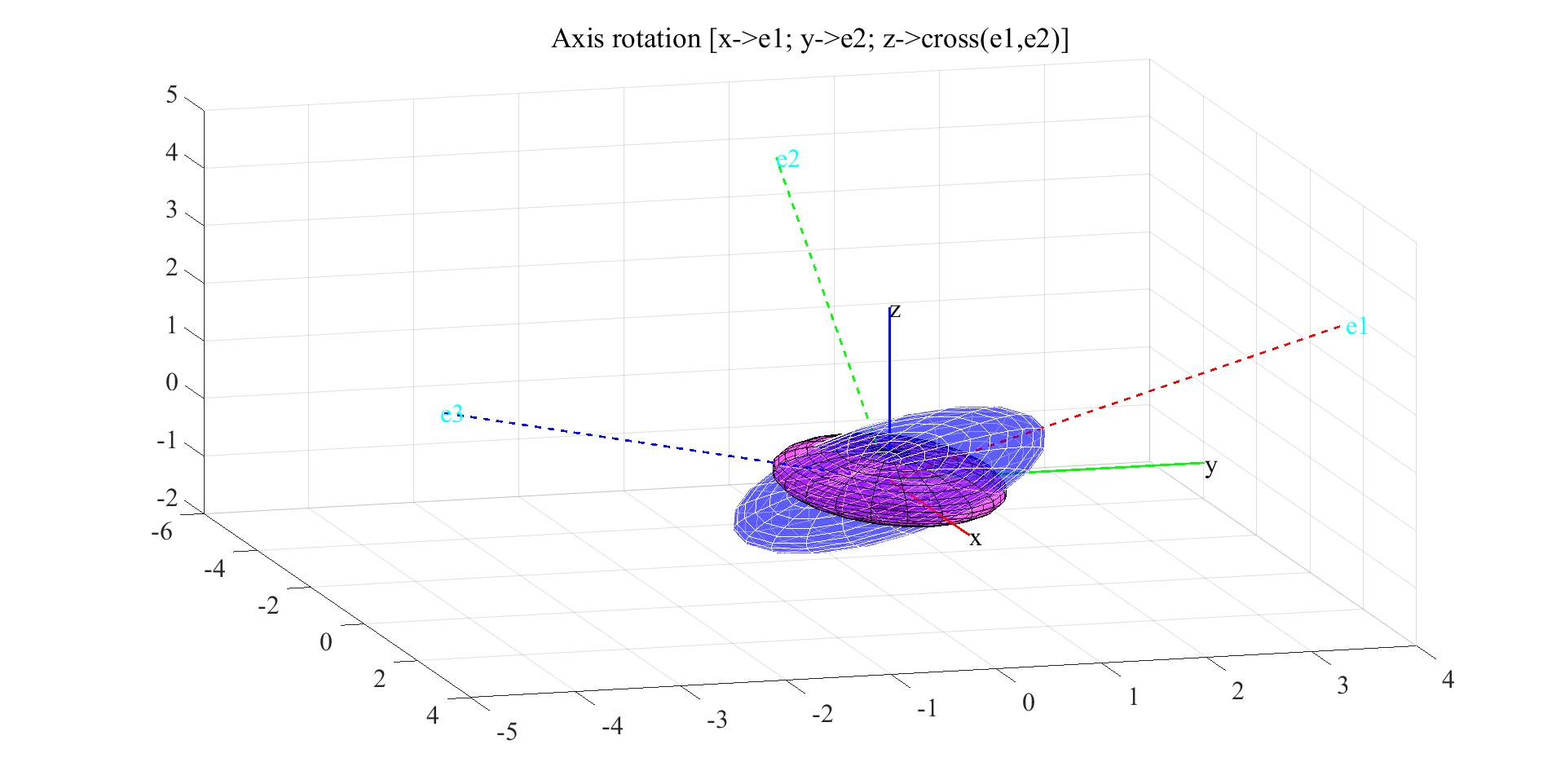## Rotate 3d object by local coordinate axis of two vectors

Version 1.0.0 (1.91 KB) by
This function is for rotating an object by two perpendicular vectors. I rotate the x-axis to e1 vector direction and y-axis to e2 vector dir

Updated Sun, 22 Aug 2021 11:59:34 +0000

This is a demo showing how to rotate an ellipsoid to a given local axis (depicted by two perpendicular vectors e1 and e2)
% x-axis to e1
% y-axis to e2
% z-axis to e3
% Coded by Wan Ji from Wuhan University
% 2021/08/22
P0 = [0,0,0]; % point origin
P1 = [3,0,0]; % point on x-axis
P2 = [0,3,0]; % point on y-axis
P3 = [0,0,3]; % point on z-axis
%% two perpendicular vectors for rotation
e1 = [1, 1, 1]/sqrt(3); % e1 vector
e2 = [-1, 0, 1]/sqrt(2); % e2 vector
%% Draw 3D axis
figure(1); title('axis rotation [x to e1; y to e2; z to cross(e1,e2)]')
plot3([P0(1), P1(1)], [P0(2), P1(2)], [P0(3), P1(3)],'r-','linewidth',2)
hold on
plot3([P0(1), P2(1)], [P0(2), P2(2)], [P0(3), P2(3)], 'g-','linewidth',2)
plot3([P0(1), P3(1)], [P0(2), P3(2)], [P0(3), P3(3)],'b-','linewidth',2)
text(P1(1), P1(2),P1(3),'x')
text(P2(1), P2(2),P2(3),'y')
text(P3(1), P3(2),P3(3),'z')
P1_rot = rotate3d(e1, e2, P1)*2;
P2_rot = rotate3d(e1, e2, P2)*2;
P3_rot = rotate3d(e1, e2, P3)*2;
%% Draw 3D axis after rotation
plot3([P0(1), P1_rot(1)], [P0(2), P1_rot(2)], [P0(3), P1_rot(3)],'r--','linewidth',2)
hold on
plot3([P0(1), P2_rot(1)], [P0(2), P2_rot(2)], [P0(3), P2_rot(3)], 'g--','linewidth',2)
plot3([P0(1), P3_rot(1)], [P0(2), P3_rot(2)], [P0(3), P3_rot(3)],'b--','linewidth',2)
text(P1_rot(1), P1_rot(2),P1_rot(3),'e1','color','c')
text(P2_rot(1), P2_rot(2),P2_rot(3),'e2','color','c')
text(P3_rot(1), P3_rot(2),P3_rot(3),'e3','color','c')
grid on
%% Rotate An ellipsoid
[x,y,z] = ellipsoid(0,0,0,2,1,0.5);
mesh(x,y,z,'facecolor','m','edgecolor','k', 'facealpha',0.4);
xyz = rotate3d(e1,e2,[x(:),y(:),z(:)]);
xp = reshape(xyz(:,1), size(x));
yp = reshape(xyz(:,2), size(y));
zp = reshape(xyz(:,3), size(z));
mesh(xp,yp,zp,'facecolor','b','edgecolor','w', 'facealpha',0.4);### Cite As

Wan Ji (2023). Rotate 3d object by local coordinate axis of two vectors (https://www.mathworks.com/matlabcentral/fileexchange/98049-rotate-3d-object-by-local-coordinate-axis-of-two-vectors), MATLAB Central File Exchange. Retrieved .

##### MATLAB Release Compatibility
Created with R2020a
Compatible with any release
##### Platform Compatibility
Windows macOS Linux